# Concept 11 Points Lines and Planes FiveMinute Check

• Slides: 32Concept 11 Points, Lines, and PlanesFive-Minute Check CCSS Then/Now New Vocabulary Key Concept: Undefined Terms Example 1: Name Lines and Planes Example 2: Real-World Example: Model Points, Lines, and Planes Example 3: Draw Geometric Figures Example 4: Interpret DrawingsContent Standards G. CO. 1 Know precise definitions of angle, circle, perpendicular line, parallel line, and line segment, based on the undefined notions of point, line, distance along a line, and distance around a circular arc. Mathematical Practices 4 Model with mathematics. 6 Attend to precision.You used basic geometric concepts and properties to solve problems. • Identify and model points, lines, and planes. • Identify intersecting lines and planes.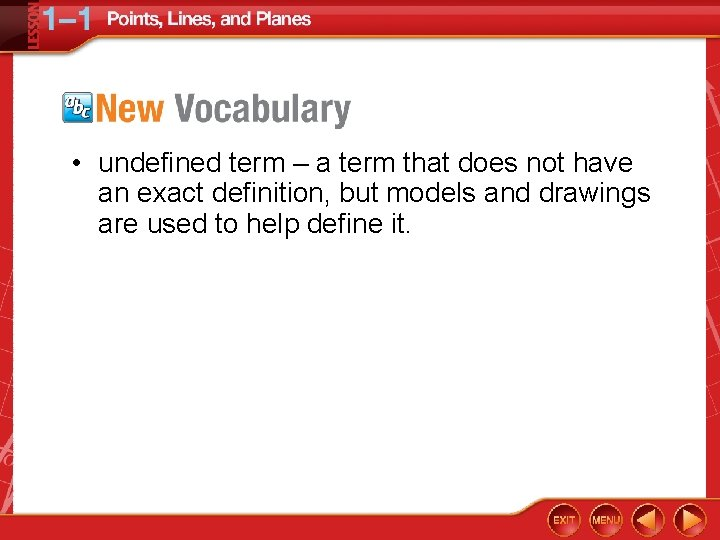• undefined term – a term that does not have an exact definition, but models and drawings are used to help define it.Name Lines and Planes 1. Use the figure to name a line containing point K. Answer: The line can be named as line a. There are three points on the line. Any two of the points can be used to name the line.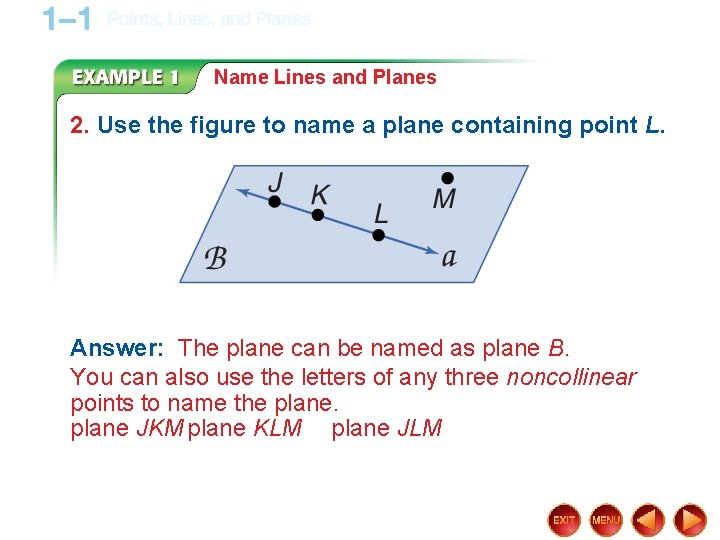Name Lines and Planes 2. Use the figure to name a plane containing point L. Answer: The plane can be named as plane B. You can also use the letters of any three noncollinear points to name the plane JKM plane KLM plane JLM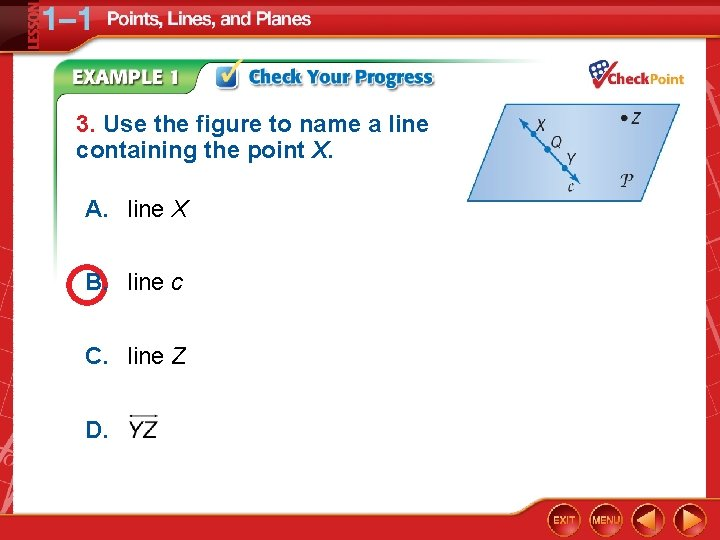3. Use the figure to name a line containing the point X. A. line X B. line c C. line Z D.4. Use the figure to name a plane containing point Z. A. plane XY B. plane c C. plane XQY D. plane P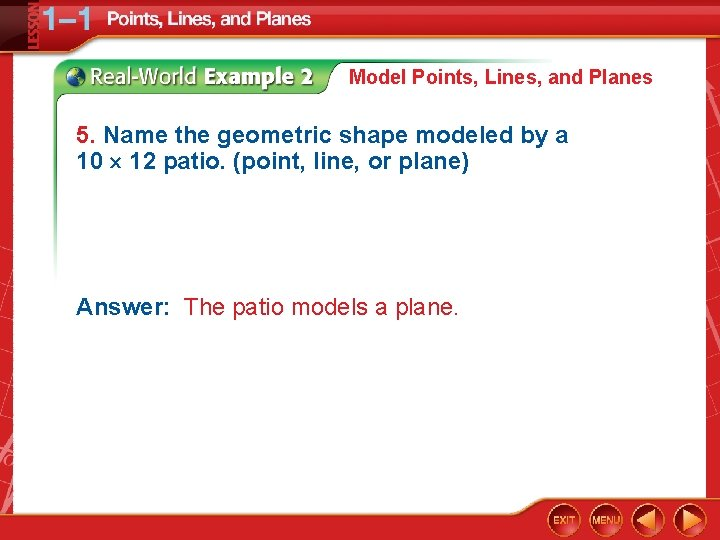Model Points, Lines, and Planes 5. Name the geometric shape modeled by a 10 12 patio. (point, line, or plane) Answer: The patio models a plane.Model Points, Lines, and Planes 6. Name the geometric shape modeled by a button on a table. Answer: The button on the table models a point on a plane.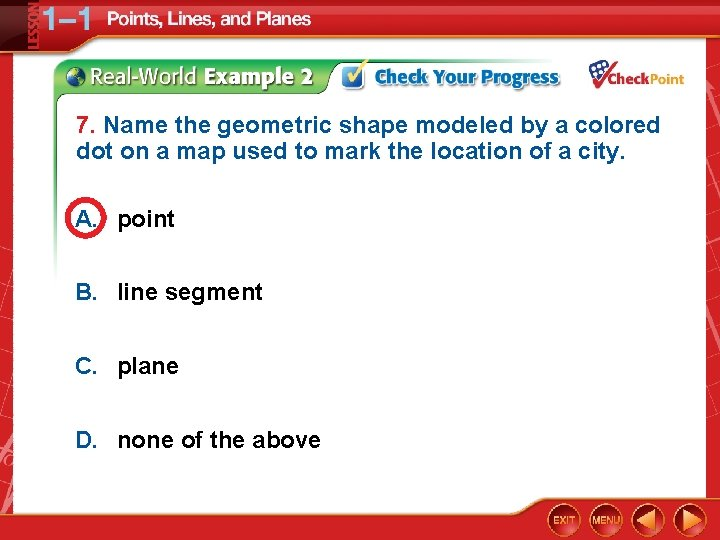7. Name the geometric shape modeled by a colored dot on a map used to mark the location of a city. A. point B. line segment C. plane D. none of the above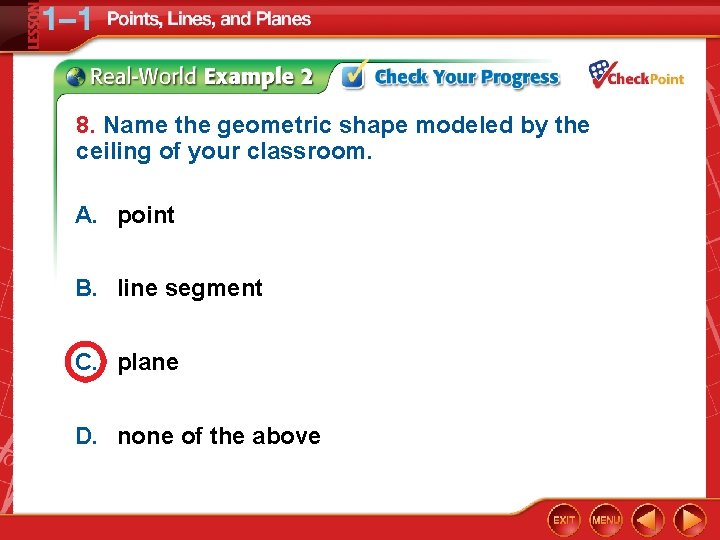8. Name the geometric shape modeled by the ceiling of your classroom. A. point B. line segment C. plane D. none of the above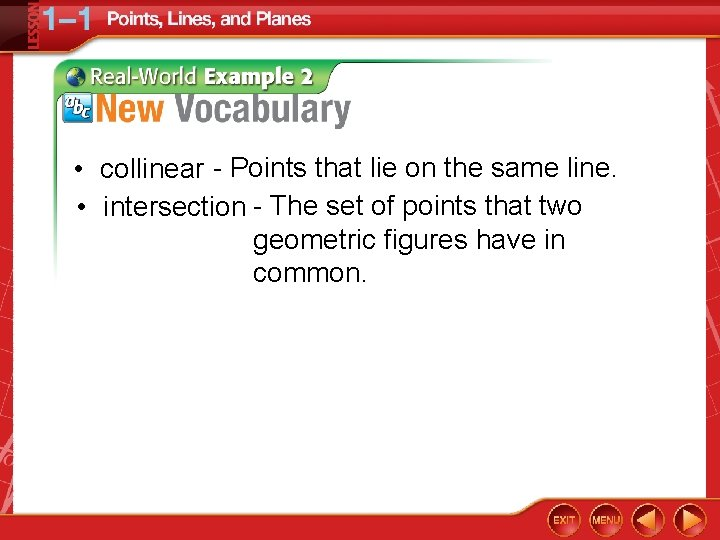• collinear - Points that lie on the same line. • intersection - The set of points that two geometric figures have in common.Draw Geometric Figures 9. Name 3 points that are collinear. 10. Is point C collinear with points A and B? 11. What is the name of the plane drawn?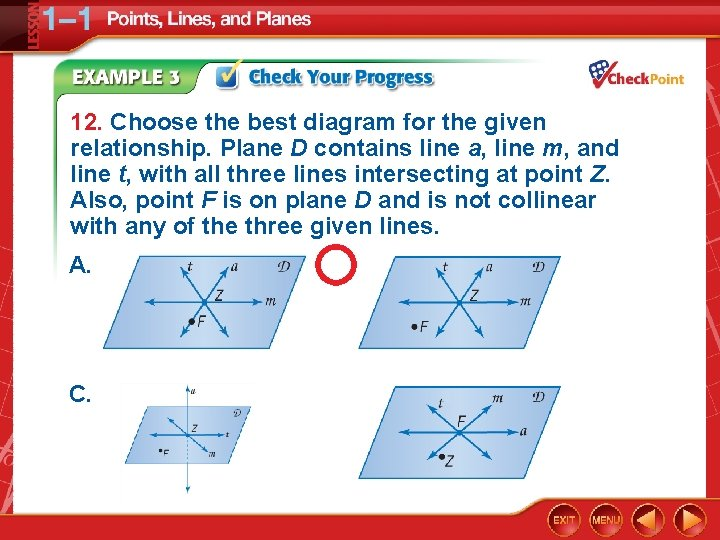12. Choose the best diagram for the given relationship. Plane D contains line a, line m, and line t, with all three lines intersecting at point Z. Also, point F is on plane D and is not collinear with any of the three given lines. A. B. C. D.Lines Can Be: • Parallel – lines that lie in the same plane and never cross. • Intersecting – lines that cross through each other and are in the same plane. • Perpendicular – lines that cross to form a right ange and are in the same plane.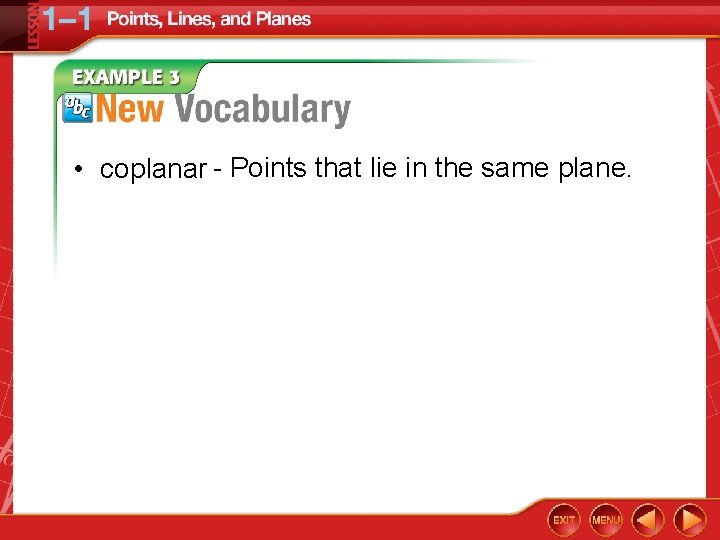• coplanar - Points that lie in the same plane.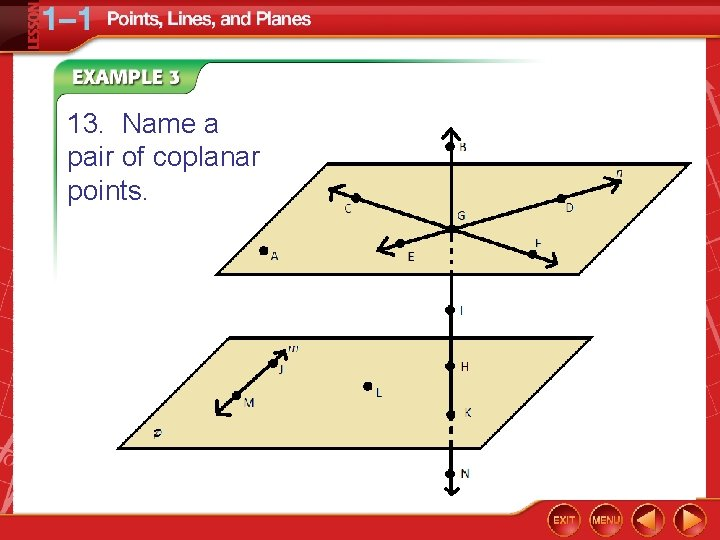13. Name a pair of coplanar points.14. Name a pair of coplanar lines.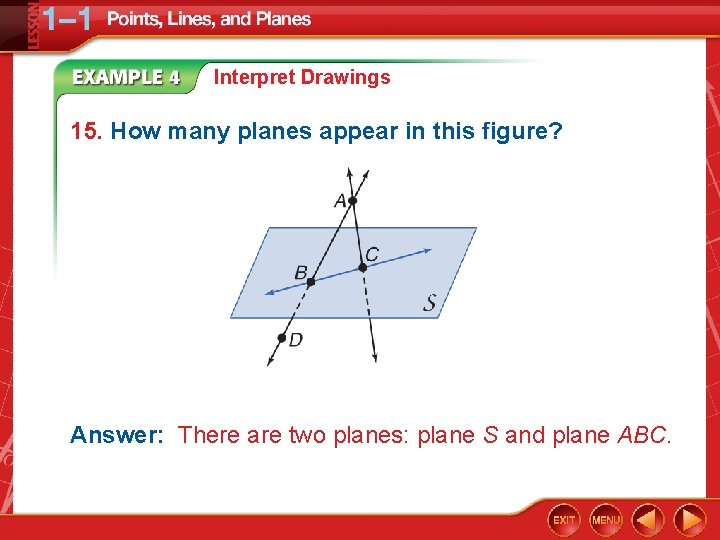Interpret Drawings 15. How many planes appear in this figure? Answer: There are two planes: plane S and plane ABC.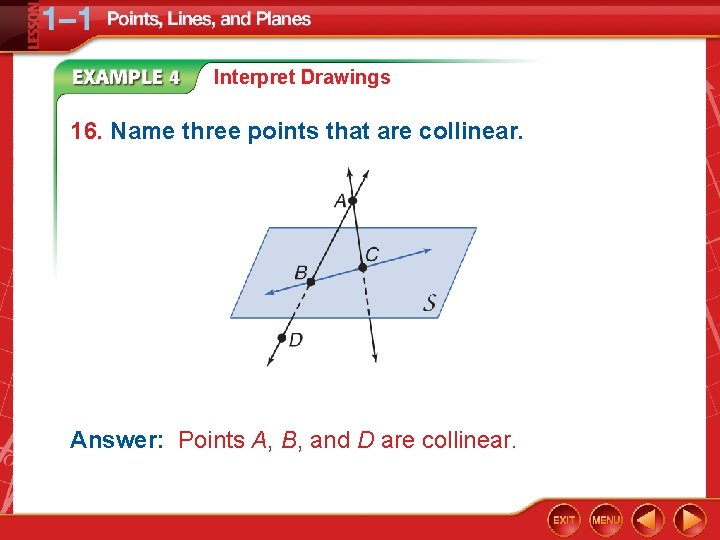Interpret Drawings 16. Name three points that are collinear. Answer: Points A, B, and D are collinear.Interpret Drawings 17. Are points A, B, C, and D coplanar? Explain. Answer: Points A, B, C, and D all lie in plane ABC, so they are coplanar.Interpret Drawings 18. Answer: The two lines intersect at point A.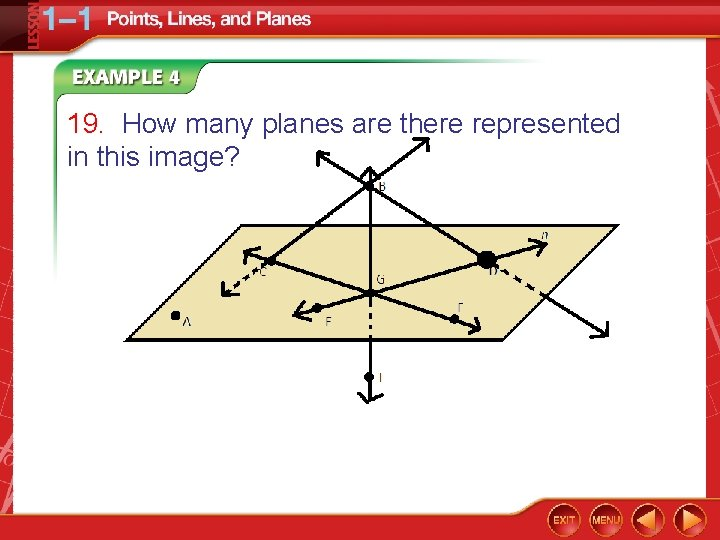19. How many planes are there represented in this image?19. How many planes are there represented in this image?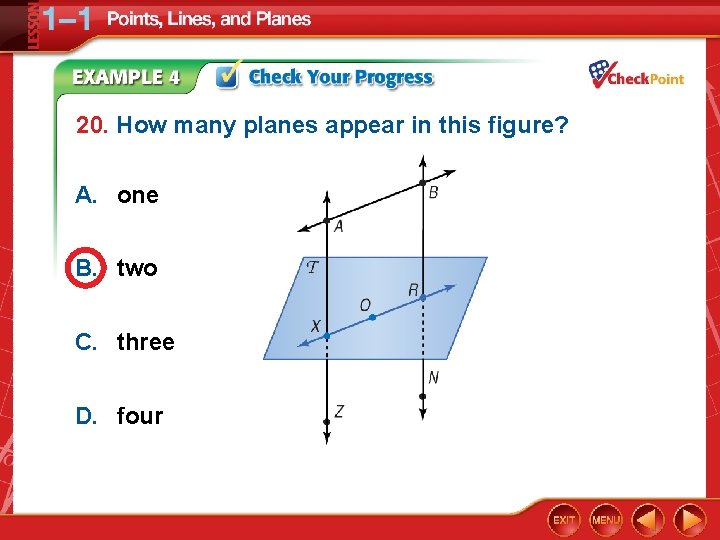20. How many planes appear in this figure? A. one B. two C. three D. four21. Name three points that are collinear. A. B, O, and X B. X, O, and N C. R, O, and B D. A, X, and Z22. Are points X, O, and R coplanar? A. yes B. no C. cannot be determined23. A. point X B. point N C. point R D. point A